## Sunday, July 19, 2015

### A Maths Problem That Could Be Solved Only by IAS Topper (2014) Gaurav Agarwal! (Updated)

This question is popping up on smartphone and tablet screens a lot lately.Since UPSC (and especially the ocean-like syllabus that you have to cover) really gets me excited,I decided to share my own way of answering this question.I am sure some of them have solved it really well,other than Gaurav Agarwal but I would be pleased to answer this on my own!

My Solution (This is truly original and I can guarantee that you can't find a replica of this answer online.) : -

1 fortnight + 15 days + 1 day = 14 days + 15 days + 1 day = 30 days

Update on 04th July,2015 : I have received two comments on whatsapp-forwards.blogspot.com
claiming that the solution given 'is not Maths'.Well,not to mention none of the commentators of course,could solve the problem but they had their comments to aid them nonetheless.And though there are several questions which do not have conventional answers in IAS.
For example,take this question (credit goes to gpuzzles),I know a way(correct) by which
adding 4 to 10 will give 2 ?
You have to add 4 hours to 10'o clock to get the answer and instead if you apply lame methods like bringing out a minus sign out of nowhere,which is what is the given solution on the mentioned website,I am sure UPSC is not going to appreciate you much.
An alternative solution (which is more mathematical in nature) can be 3! + 13 + 11 = 30 but from where do you get the factorial sign is the counter-question.Nonetheless,I think the solution with 1 fortnight is substantial and authentic than lame methods of bringing mathematical signs out of nowhere.

Update on 19th July : Here is an alternative solution from Jagadish Ramanna,who is a M.Tech student at East West Institute of Technology,Bangalore.
We have  x + y + z = 30 (Considering x,y,z to be random variables.)
Let x+y+z=30
then x+y+z=30 implies
x+y+z-30= 0
divide whole by 2
(x/2) + (y/2) + (z/2) - 15 = 0
let x=9 , y =3 , z= 3 ( prime numbers given )
(9/2) + (3/2) + (3/2) = 15
(9 + 3 + 3)/2 = 15
therefore
9+3+3 = 30
Hence solved ﻿

we can take x,y,z as 9,3,3 or 11,1,3 or 9,5,1 or 13,1,1

A Little Additive : The post has received quite a number of Plus Ones on Google+ and the blog is receiving traffic everyday on searches related to Gaurav Agarwal.I have even had a conversation on Twitter from a previously totally unknown person about this particular problem,it was upvoted several times on IndiBlogger and the second version including 5 blanks instead of 3 was also very well received.I hope that does a make it a little authentic from the perspective of public verdict.

We have reached 9,000+ reads on this post.Can we get it to 10,000? This would help in reinstating my interest in UPSC problems. ;)
Thank You so much for helping us reach 50,000 hits on this post!

1.Assumption let x=9 , y =3 , z= 3 ( prime numbers given )
Doesn't comply with above equation...

1.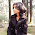Hi there,
Well,you can't really answer it with arithmetic logic.I think it is logically sane.Might be you would like to go by the first alternative.

2.also 9 is not a prime number, any school student will know

3.Given is an equation not an identity to give values you like

4.Given is an equation not an identity to give values you like

5.But, Let x+y+z=30
x+y+z-30=0 divide whole by 10
x/10+y/10+z/10-3=0
Let x=1, y=1, z=1
1/10+1/10+1/10=3
Therefore 1+1+1=30
// so that we can also take x,y,z as 1,1,1.

6.NO NO NO..... ITS ALL WRONG.. one box means on number should be entered.. rather to use formulas in it....

7.NO NO NO..... ITS ALL WRONG.. one box means one number should be entered.. rather to use formulas in it....

2.One response is 15+7,5+7,5=30

1.Hi,
Can you explain that solution a little further?

2.He is native French speaker, so 7,5 for him is 7.5 for English speakers. The separation of thousands in French is a space, so 7,500.5 in English is 7 500,5 in French.

But the question was in India an English speaking country so his solution won't be accepted there.

3.My answer is to suppose we are using Octal, not decimals numbers, than 15+13=30.
No need to put anything in the last square.

4.My answer is to suppose we are using Octal, not decimals numbers, than 15+13=30.
No need to put anything in the last square.

5.If you want to use the decimal one than, you just repeat 15,then 15+15=30. Again, no need to put anything in the last square.

3.The answer provided is simply cheating. It is quite a very simple demonstration (simplified to three numbers):
n1 + n2 + n3 = n4' where n1, n2, n3 are odd and n4' even.
all odd numbers can be written n1 = n1' + 1, where n1' is even, so
n1' + 1 + n2' + 1 + n3' + 1 = n4'
n4' - 3, being n4 even, is always an odd number, so
n1' n2' + n3' = n4
every even number can be decomposed by the sum of another even number plus 2, so
2 x (n1' + n2' + n3') = n4
Being n4 an odd numer it can not be divisible by 2 and therefore the equivalence is not correct.
Cheers!

1.But the compulsion in the question is to trickily answer it,not to determine if the equivalence is right or wrong.We all know that conventionally solving this is not possible.

4.Write the 9 upside down so it becomes a 6 (it doesn't say how the symbols can be written in the boxes) 6+11+13

1.This is cool! :)

2.Or add a ! and use factorials to do 13+11+3!=30 (3!=6)

5.I think we have to use the two parenthesis and all the numbers:

Box#1: using 5 and 3 as a binomial coefficient = (5 3); according to binomial coefficient (5 3) =5!/(3! (5 -3)! = 5!/(3!×2!)=5×4×3!/(3!×2!) = 5×4/2! = 10

Box#2: 11

Box#3: 9

(5 3) + 11 + 9 = 30

Cheers!!!!

1.But are we allowed to use parenthesis inside the boxes? Otherwise, this is interesting!

6.This comment has been removed by the author.

7.well ... sometime i wrote number "1" as just a line ... like "|"

so ... maybe can i use that | as "1" into the following ??

 +  + [|-|]
15 + 15 + 0 = 30

:D

1.Trickkyy! :P

8.(9 + 3 + 3)/2 = 7.5. Not 15

1.Conventionally,you can't solve this.Because odd + odd + odd = odd,not even.Please re-read the second solution.The trick is right in there and valid.

9.11(10)+5(10)+5(10)=30(8)
i.e. 11 (base 10) + 5 (base 10) + 5(base 10)= 30 (base 8) = 21 (base 10)

A.Armengol C.

1.Wow.That's really substantial.

10.Well, there's also this:

log (base 3) from 9 =2 ---> so I will write it like this log(3,9)
log(3, 9) + 13 + 15 = 30

11.7.9+9.11+13= 30.01 ~30

Or
9.5+9.5+11=30

Mayss Alsafi & Amir Murib

1.Great method! This is a substantial possibility.

12.we can write any single number as:
2n+1 or 2s+1 or...
and any even number as:
2m or 2z or...
the numbers in the problem are single, so we can write the equation as follow:

(2m+1)+(2z+1)+(2s+1) =2m+2z+2s+2+1=2(m+z+s+1)+1

m, z and s are nature numbers so we can take m+z+s+1 as a nature number too and call it k so we have:

2(m+z+s+1)+1=2k+1
it is means three single numbers with each other never can give us a even number so tge broblem cant be solve under the given condition!

1.No,I think the solution has to be TRICKY.That is the case with several problems that are asked by UPSC in Indian Administrative Service examinations.

13.Well, it says fill in the boxes but not all the boxes...
So 15+15+(box)=30 :p

1.14.(9*3)+(3)+(1-1)=30
(5){+(3)+(3)}=30

1.Not sure if we can use multiple numbers in a single box.

15.This problem has no solution

1.What are tricks and gaps made for,then?

16.the base of numbers is not mentioned so try this for base 11; 3+13+15 = 30 am i correct?

1.Yes...if we can use base.

2.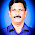This comment has been removed by the author.

3.This comment has been removed by the author.

17.why you people think always about decimal system only,try asolution for other bases greater than 10; EX. for base 11 ,3+13+15 is exactly 30
again for base 13; 9+11+13 =30

18.Here you go
5( + 3 + 3) = 30
5 (6) = 30

it only said "Fill the boxes using "(" 1,3,5,... ")" "
so we can technically use the ( ) too.

1.19.Its to simple:

We have following Numbers:
1,3,5,7,9,11,13,15

It depends how you look at the numbers.

There is only one solution, and its mathicmaticaly correct. We dont know, if the commas seperate the numbers, or if the comma belong to the specific number.
It could be 1,3 and 5 and 7...
It could be 1 and 3 and 5,7 and 9...

But the only possibility is the following:

1 and 3 and 5 and 7 and 9 and 11 and 13,15

So the right answer without any crazy story or deep mathematic formulas is following:

3 + 13,5 + 13,5 = 30

Thank me later bitches.

1.No one's thanking you yet.This is nothing original.

20.Create zero using two parenthesis(well, () make this more close to each other), then the solution is 0+15+15. We can use the parenthesis, since in the question they are saying "using the following"

21.Create zero using two parenthesis(well, () make this more close to each other), then the solution is 0+15+15. We can use the parenthesis, since in the question they are saying "using the following"

1.They didn't provide parenthesis as an option to fill the box with.

22.3+13+15=30 in base 9
In base 9
3+14+16=33

1.This comment has been removed by the author.

2.This comment has been removed by the author.

3.This comment has been removed by the author.

23.(13)+(15)+(1+1)=30
or
(13)+(15)+(9-7)=30

24.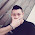My number is 15+15+NO=30

25.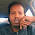26.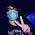Well, the answer can also be:

15+9+3!=30 (Where '!' is the factorial function i.e. 3! = 3x2x1 = 6)

1.That's the one that jumped at me :p

27.15 1/3 + 9 1/3 + 5 1/3 = 30

28.This comment has been removed by the author.

29.Take 13 as a number with base 7 so that it is 10 in the decimal system. Take 30 with base 10.

30.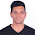This comment has been removed by the author.

1.This comment has been removed by the author.

31.The question is to fill the boxes using (1,3,5,7,9,11,13,15) & has not specified 'using the numbers'. So I guess the two paranthesis provided in the question can be used.
So, solution is (5 3)+5+15 = 30,
where (5 3) represents binomial coefficient, 5C3 = 10.

32.++

It might be help :)

1.Kidding dude. So frustrated here lol

2.Easy one 15+15+(1-1)=30

33.VOCÊ CONSEGUE RESOLVER ESSA? Resposta:NÃO A soma de três números impares, nunca terá como resultado um número par.

34.+  +  = 30 and  +  +  = 30 by chromedkim

35.Box 1: (1
Box 2: 1)(
Box 3: 15)

So it becomes (1 + 1)(+15) = =30
(2)(15) = 30
2 × 15 = 30
30 = 30

Solved!!

36.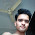3!+11+13=6+11+13=30

37.Put 26 (thirteen repeated once) in one box, 3 in a second box, and 1 in your remaining box. Only thing I could think of that I hadn't heard in a post yet.

38.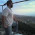Assumption let x=9 , y =3 , z= 3

You are aware that you are a complete idiot, arent you? :)
you put just random numbers and you get a wrong answer where 15 = 30 and you consider that hence you solved the world'd problems?

If you are over 12 years old, you ARE a complete idiot.

Best Regards,

1.Kindly control your hormonal balance and have a look.The question says 'tricky'.

Regards,
The Perspectives

39.Let x+y+z=30
then x+y+z=30 implies
x+y+z-30= 0
divide whole by 2
(x/2) + (y/2) + (z/2) - 15 = 0
let x=9 , y =3 , z= 3 ( prime numbers given )
(9/2) + (3/2) + (3/2) = 15
(9 + 3 + 3)/2 = 15
therefore
9+3+3 = 30
Hence solved ﻿

we can take x,y,z as 9,3,3 or 11,1,3 or 9,5,1 or 13,1,1

40.(11-1)+(13-3)+(15-5)=30

41.(11-1)+(13-3)+(15-5)=30

42.(11)+(13)+(3!)=30